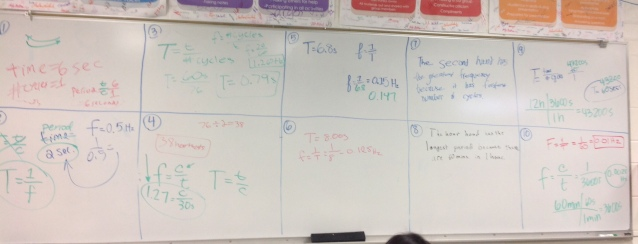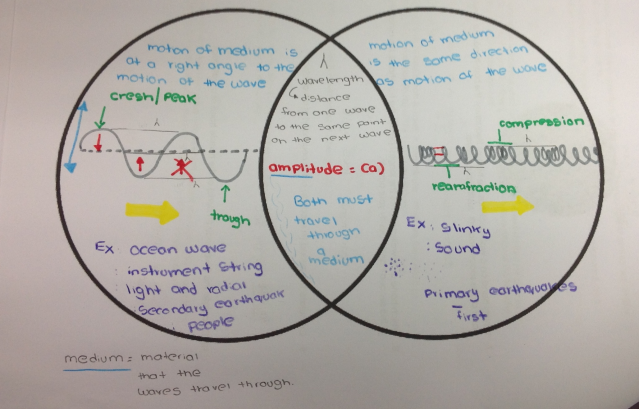# Periodic Motion and Waves

2 teachers like this lesson
Print Lesson

## Objective

Students will be able to recognize two types of periodic motion (transverse and longitudinal waves) and label the appropriate parts of each type of wave.

#### Big Idea

Students identify longitudinal and transverse waves as a type of periodic motion.

## Pendulum Lab Discussion

10 minutes

The goal of this lesson is for students to understand how period and frequency are related and to calculate those quantities. Students discuss the results of their lab and engage in evidence supported arguments on what relationships exist (SP7, SP8). This helps students as they work through the simulation to develop a model for other relationships eventually leading to HS-PS4-1. Students are also introduced to the different types of waves and begin to investigate the properties of transverse waves through a simulation. Finally, students use skills of computational thinking and developing models throughout the notes and the simulation (SP2).

To start out class, I want to wrap up the Pendulum Lab that students completed in the previous lesson with a discussion. I ask students to take out their labs and I ask them how they answered the questions about the relationships between frequency and mass, length and amplitude. We discuss each relationship by students volunteering their data to support their answers. As we go over each relationship, I ask students to look at the predictions that they made before the lab to see if their predictions were correct.

## Mega-Whiteboard of Period and Frequency Practice Problems

15 minutes

After we finish discussing the lab, I ask students to turn to the homework that they did on the Period and Frequency WS. To go over this worksheet, I randomly select 9 students from my list of index cards, each with a student name on it. The students that are selected put their work to a problem up on the whiteboard for a mega-whiteboard session.After students put their work on the board, I read the question and ask students if they agree with the work and answer that is on the board. If they don't agree, I tell them that they need to ask a question. I go over these problems this way because the period and frequency equations are pretty straightforward so students usually can look through the answers pretty quickly and get most of the answers correct. You can see on a sample of one student's complete worksheet, shown below, the work that I am expecting for these types of problems.## Period and Frequency Checkpoint

5 minutes

After going over the worksheet answers, I want to see what students can do on their own with a quick checkpoint on Socrative. Socrative is an online tool that I use to assess students that gives immediate feedback to students of their individual progress as well as to me of student's overall progress. The students log-in to the site on their Chromebooks using my room code that I put on the board and complete the four questions on the Period and Frequency Checkpoint. After students are done, I use this to just gauge whether I can move on or not.

## Intro to Waves Notes

20 minutes

After the checkpoint, I ask students to take out their packets and turn to the Intro to Waves Venn Diagram. I use a Venn Diagram for these notes because we are comparing and contrasting the different types of waves: transverse and longitudinal. As seen in the picture and the video below, I start out with drawing the different types of waves and labeling the parts of each wave. I make sure to note that wavelength and amplitude are present in both types of waves. Then we move onto how each wave moves through a medium and how it is different for each type of wave. Finally, we end with coming up with some examples of each type of wave as seen at the bottom of this student's Venn Diagram shown below.## PhET Simulation: Wave on a String

20 minutes

After the notes, I have students turn to the PhET Simulation Activity. Since we don't have much time left in class, I just have students start the activity. This activity gives students a chance to investigate the relationships of different properties of a wave based on the Wave on a String simulation. They have never seen this activity before so I help students set up the simulation and work through Part 1.

Students use their chromebooks to open this simulation. Recently they have added an html version which is more user friendly that I will be using next year for students. When we set up the simulation, I make sure that each student has his or her system on oscillate with no end. Then I ask students to make sure the rulers and timer are checked so we can use those tools. Finally, I ask students to move the damping dial to 0. Their screen should match the screen that I am projecting which is shown below.After each student has confirmed that his or her screen matches mine, I direct students to look at Part 1: Does amplitude affect wavelength? I help students move through this part step by step, based on the instructions in the PhET Simulation Activity, so that they can see how to work the simulation. The students end up finding that there is no relationship between amplitude and wavelength with the data shown below.To end class, I ask students to review the relationships that we know know between period and frequency and amplitude and wavelength so students recall what they have learned for the day.